Next: Exercises Up: Probability Theory Previous: Mean, Variance, and Standard

# Continuous Probability Distributions

Suppose, now, that the variable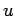can take on a continuous range of possible values. In general, we expect the probability thattakes on a value in the rangetoto be directly proportional to, in the limit that. In other words,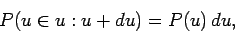(23)

whereis known as the probability density. The earlier results (5), (12), and (19) generalize in a straightforward manner to give(24)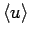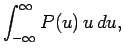(25)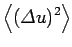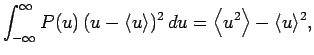(26)

respectively.

Subsections

Richard Fitzpatrick 2010-07-20